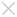English

# Happy CADing with ZW3D: Design a Delicate XO Bottle

April 22, 2015     Happy CADing Design a XO Bottle

Getting together with friends to have a little chatting and drinking is the happiest thing. These delicate and beautiful glass bottles, you can’t put them down.

For a CAD designer, the more interesting thing is how to design this kind of attractive bottle, as shown in Figure 1. Today, you will learn more from this article.Figure 1: XO Bottle

Step 1: Insert the sketch1 on XZ plane, and then draw the half of ellipse with 160*110 as shown in the Figure 2(the left one).

Step 2: Create a new datum1 by offsetting the XZ plane 16mm. Insert the sketch2 on this new datum, and then draw the half of ellipse with 100 * 80; see Figure 2(middle).

Step 3: Mirror the sketch2 with XZ plane; see Figure 2(right).Figure 2: Draw the sketches

Step 4: Select the YZ datum to draw the sketch3. The reference point definition is illustrated by the following image. Then use the point curve command to draw the curve. The start point and end point need to be added tangent constraint.Figure 3: Draw the profile sketch

Step 5: Select the XY datum to draw the sketch4. Define the reference point and then draw the arc; see Figure 4(left). After that, all the profile sketches are ready; see Figure 4(right).Figure 4: Profile sketches

Step 6: Create the surface with UV curve mesh command; see Figure 5.Figure 5: UV curve surface

Step 7: Draw the sketch5 on XZ plane (see Figure 6).Figure 6: Draw the sketch5

Step 8: Split the surface with the sketch curve, and then delete redundant parts to keep the small surfaces, as shown figure7.Figure 7: Split the surface

Step 9: Draw the sketch 6 on XZ plane and draw the sketch7 on datum1. And mirror the sketch 7.Figure 8: Sketch6 & 7

Step 10: Use the UV curve mesh tool to create the surface. Sew all surfaces together, figure 9 (right).Figure 9: UV curve surface

Step 11: Draw the sketch 8 on the XZ datum, as shown in the figure 10 (left). And then extrude the sketch from 6mm to -8mm. Set the draft angle as 16 degree.Figure 10: Create the bottle base shape

Step 12: Draw the sketch9 on XZ datum, as shown in figure11 (left). Do the extrude operation to cut the bottle base shape; see figure11 (right).Figure 11: Extrude cut the base shape

Step 13: Draw the sketch10 on XZ datum, as shown in figure12 (left).And then Extrude the sketch to get a surface; see figure12 (right).Figure 12: Create the trimming surface

Step14: Use this surface to trim the base shape and another surface.Figure 13: Trim shape and surface

Step15: Create the sketch11 as shown in figure14 (left). Project this sketch to the top surface of base shape; see figure14 (right).Figure 14: Project curves to face

Step 16: Use the projected curve and face edges to define the FEM patch. Refer to the following image to define them.Figure 15: Create surfaces with FEM patch

Step 17: Add the fillet (0.5mm) on the base shape. Add the fillet (3mm) on the body of bottle.Figure 16: Fillet

Step 18: Draw the sketch12 on XZ datum, as shown in figure17 (left).Revolve it and combine two shapes together, and then add the fillet (1mm). The final result is shown below.Figure 17: Revolve the sketch

Step 19: Shell the shape with the 1mm thickness. And then, define the top face as open face.Figure 18: Shell the shape

Step 20: Create two new datum planes by offsetting XY datum to 96mm and 104mm; and create two octagon sketches. The diameters of out circle respectively are 20mm and 12.6mm; see figure 19.Figure 19: Create the loft profile

Step 21: Use loft command to create the surface. One point should be noted that the directions of profiles should be same.Figure 20:Loft the surface

Step 22: Use the 3 points to define a new plane. And then make a pattern as shown in the figure21 (right).Figure 21: Create the datum planes

Step23: Use these datum planes to trim the shape. And then create a sketch to get a revolved shape. Finally, add the suitable fillet features on the shape.Figure 22: Design process of bottle stopper

Step24: Design the logo as you like.Figure 23: XO Bottle Shape

How to use ZW3D quickly create a product? You can get a general idea from this case. From datum plane, sketch to solid and surface design, unique solid-surface hybrid modeling technology helps you to quickly do product design. Many good surface tools are provided, such as: loft, UV curve mesh, FEM patch.  ZW3D designs your idea.